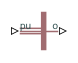# Induction Machine Measurement

Per-unit measurement from induction machine

•Libraries:
Simscape / Electrical / Electromechanical / Asynchronous

## Description

The Induction Machine Measurement block outputs a per-unit measurement associated with a connected Induction Machine Squirrel Cage or Induction Machine Wound Rotor block. The input of the Induction Machine Measurement block connects to the `pu` output port of the induction machine block.

You set the Output parameter to a per-unit measurement associated with the induction machine. Based on the value you select, the Induction Machine Measurement block:

• Directly outputs the value of an element in the input signal vector

• Calculates the per-unit measurement by using values of elements in the input signal vector in mathematical expressions

The Induction Machine Measurement block outputs a per-unit measurement from the induction machine according to the output value expressions in the table. For example, when you set Output to Stator d-axis voltage, the block directly outputs the value of the pu_vds element in the input signal vector. However, when you set Output to Slip, the block calculates the slip value by subtracting the value of the pu_velocity element from 1.

Output Parameter SettingOutput Value Expression

Electrical torque

pu_torque

Rotor velocity

pu_velocity

Stator d-axis voltage

pu_vds

Stator q-axis voltage

pu_vqs

Stator zero-sequence voltage

pu_v0s

Stator d-axis current

pu_ids

Stator q-axis current

pu_iqs

Stator zero-sequence current

pu_i0s

Slip

1-pu_velocity

Apparent power

$\sqrt{pu_P{t}^{2}+pu_Q{t}^{2}}$

Real power

pu_Pt = (pu_vds*pu_ids) + (pu_vqs*pu_iqs) + 2(pu_v0s*pu_i0s)

Reactive power

pu_Qt = (pu_vqs*pu_ids) – (pu_vds*pu_iqs)

Terminal voltage

$\sqrt{pu_vd{s}^{2}+pu_vq{s}^{2}}$

Terminal current

$\sqrt{pu_id{s}^{2}+pu_iq{s}^{2}}$

power_factor_angle = atan2(pu_Qt, pu_Pt)

Power factor

cos(power_factor_angle)

## Ports

### Input

expand all

Physical signal vector associated with per-unit measurements from a connected induction machine. The vector elements are:

• pu_torque

• pu_velocity

• pu_vds

• pu_vqs

• pu_v0s

• pu_ids

• pu_iqs

• pu_i0s

### Output

expand all

Physical signal associated with the per-unit measurement output.

## Parameters

expand all

Per-unit measurement from induction machine. Options are:

• `Electrical torque`

• `Rotor velocity`

• `Stator d-axis voltage`

• `Stator q-axis voltage`

• `Stator zero-sequence voltage`

• `Stator d-axis current`

• `Stator q-axis current`

• `Stator zero-sequence current`

• `Slip`

• `Apparent power`

• `Real power`

• `Reactive power`

• `Terminal voltage`

• `Power factor angle`

• `Power factor angle`

## Version History

Introduced in R2013b